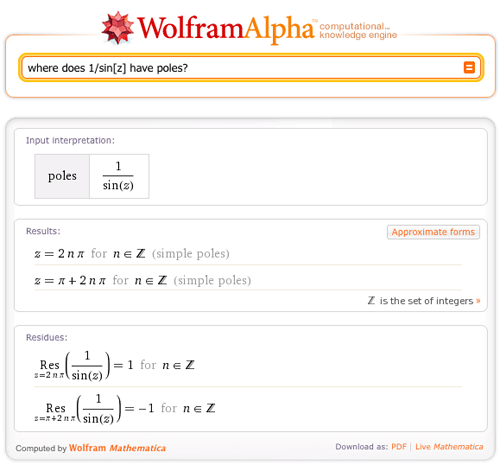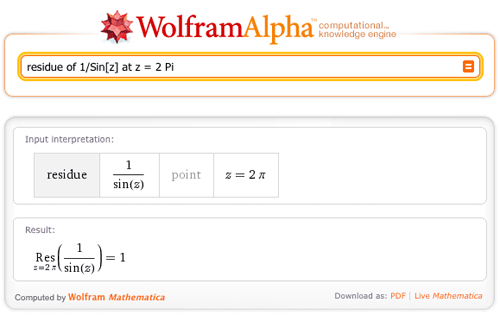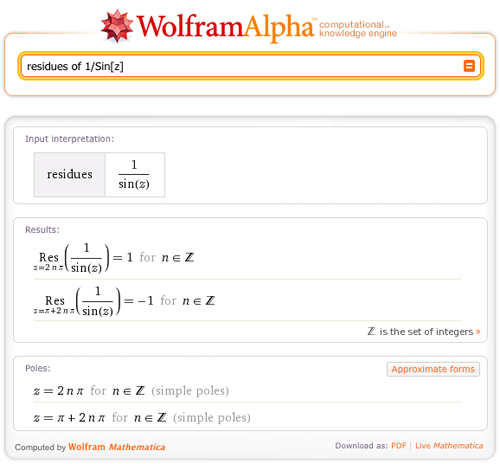# New Features for Students of Complex Analysis

February 25, 2011 —Comments Off

Why did the mathematician name his dog Cauchy? Because he left a residue at every pole!

But could the mathematician find the poles and their residues for a given function? He certainly could, with the help of Wolfram|Alpha.

We are proud to announce that Wolfram|Alpha has added residues and poles to its ever-expanding library of mathematical data that it can compute! To showcase this behavior, let’s first recall just what a pole is.

In the study of complex analysis, a pole is a singularity of a function where the function behaves like 1/zn at z == 0 .

More technically, a point z_0 is a pole of a function if the Laurent series expansion of the function about the point z_0 has only finitely many terms with a negative degree of zz_0. As an example, let’s look at the Laurent expansion of 1/Sin[z] at z == 2 Pi:

We can see here that there is only one “z – 2 Pi” term with a negative degree, and that is the term 1/(z – 2 Pi). This lets us know that 1/Sin[z] has a pole at z == 2 Pi.

Where else does this function have poles? Let’s ask Wolfram|Alpha!In complex analysis, we are particularly interested in the residue of a function at its poles. Looking at the Laurent expansion of a function about a pole, z_0, we can easily see its residue at z_0, because the residue is the coefficient of the (zz_0)-1 term.

In our example above, where we looked at the Laurent expansion of 1/Sin[z], we found that it had exactly one term with a negative exponent, and that term was 1/(z – 2 Pi). As we look at this term now, we see that the residue for 1/Sin[z] is 1. Let’s double check our work using Wolfram|Alpha:More generally, what are the residues of 1/Sin[z]?And, for fun, let’s look at a few others:

We are proud to announce that Wolfram|Alpha is now a growing resource for students of complex analysis. We hope that you enjoy exploring this new addition to Wolfram|Alpha!

Posted by George Madaraz February 26, 2011 at 2:50 pm

Okay, I’m going half crazy trying to make this work. When I type in

Res[1/z, 0]

WA tells me. But when I want to do the single most bonehead-obvious thing in the world WITH that answer, namely this:

2*pi*i*Res[1/z, 0]

WA will not do it, no matter how hard I try to make it understand. It ignores everything but the “find the residue” part, whether it’s before or after, written in words or symbols. Is there any way past this?

(Obviously I can do two separate queries, but this is a trivial example. The one I’m actually working on is much messier. If WA could remember the answer to the first one, that’d be fine too, but I type in “ans” and it asks if I mean a SPACECRAFT.)

Posted by Zeke May 7, 2014 at 11:25 pm

Thank you for your comment! This is a great suggestion and we are working on adding it!

Posted by The Wolfram|Alpha Team May 13, 2014 at 3:24 pm